## Introduction

This ad-hoc post is dedicated to one small proof from a textbook “Mathematics for Computer Science”  which is a recommended reading in the course “Formal Software Verification” . It took a while for me to understand the reasoning behind this proof so I decided to share my experience in a blog post because I did not find any information about it on the internet. Hopefully it will help someone who is working with this textbook to understand the material better.

My goal is to fill in the details I missed in the textbook, not to give a complete explanation of a problem so it is most likely that this post will only make sense to those who are familiar with the context of this proof: the chapter called “6.3 Partial Correctness & Termination”.

## A Southeast Jumping Robot

This proof is located in section “6.3.4 A Southeast Jumping Robot (Optional)”, page 180 of the book and page 188 of a textbook. It proves the following claim:

Claim 6.3.4. The robot will always get stuck at the origin.

The following is a direct quote from a textbook: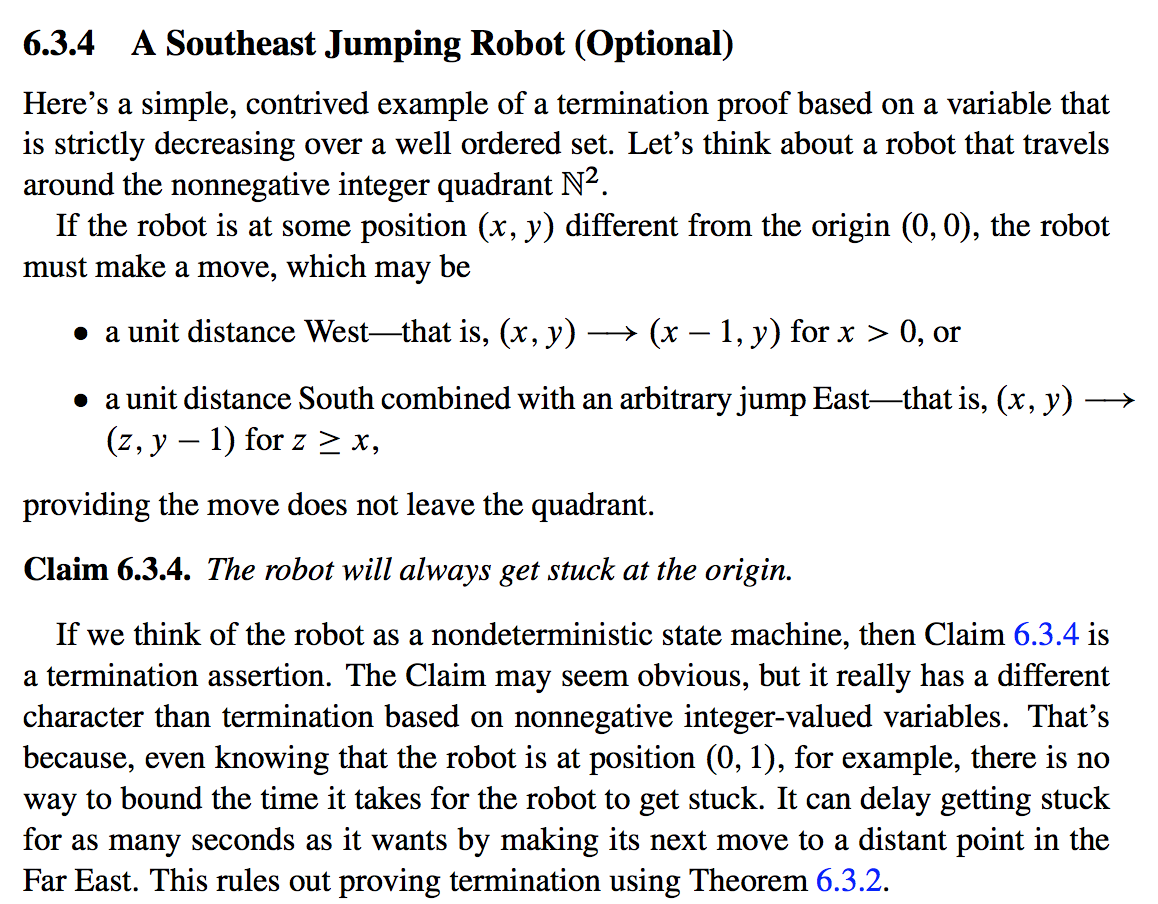## Understanding the problem

Claim 6.3.4. The robot will always get stuck at the origin.

The key observation about the behaviour of the robot is that even though the robot can jump East quicker than West, sooner or later it will not be able to jump East when it reaches the bottom of the quadrant due to the `y - 1` component of the Southeast move. After the robot has reached the bottom of the quadrant it can only move West so finally it will get stuck in `(0, 0)` because the West move will also become impossible.

## Proof

This is the proof that the textbook suggests: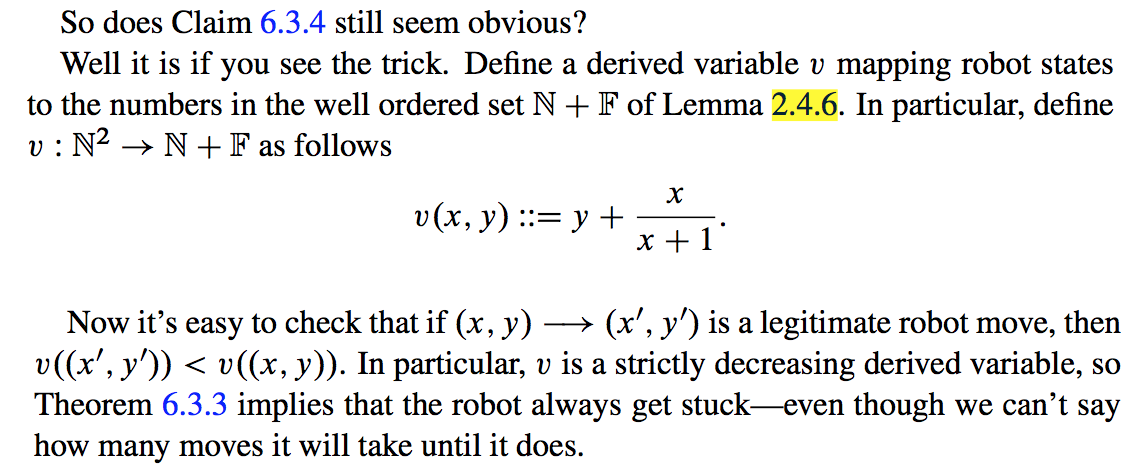Let’s consider three building blocks of this proof:

1) Lemma 2.4.6

2) Show that `𝑣` is strictly decreasing i.e. show the implication:

(𝑥, 𝑦) ⟶ (𝑥', 𝑦') ⟹ 𝑣((𝑥', 𝑦')) < 𝑣((𝑥, 𝑦))

3) Show the implication from Theorem 6.3.3 that Robot always get stuck.

### 1. Proof of the lemma 2.4.6

Let’s look the lemma up in the textbook: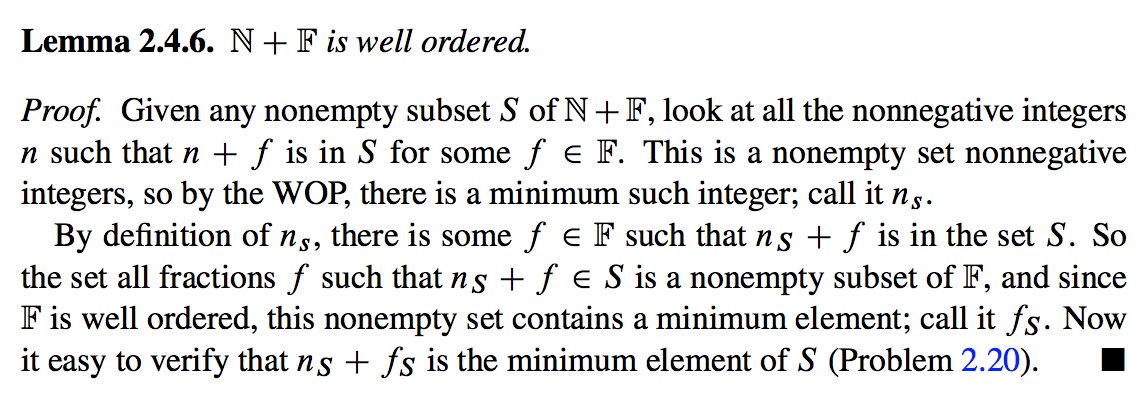Lemma 2.4.6. ℕ + 𝔽  is well ordered.

The definition of `ℕ + 𝔽` requires us to understand what is 𝔽 first: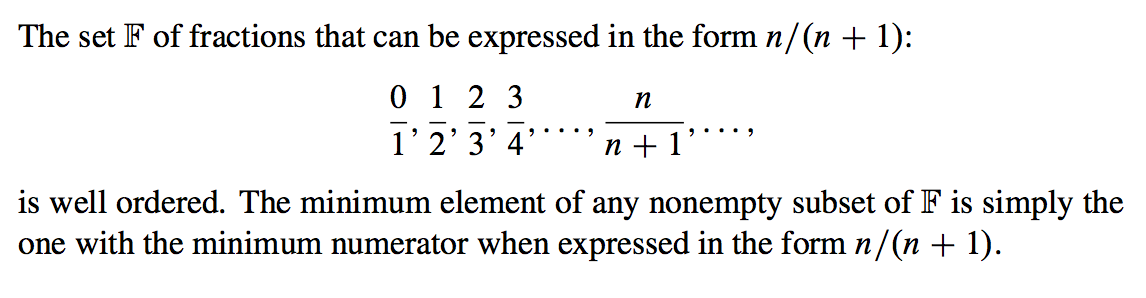This is the definition of well-ordered by Wikipedia:

In mathematics, a well-order (or well-ordering or well-order relation) on a set S is a total order on S with the property that every non-empty subset of S has a least element in this ordering. The set S together with the well-order relation is then called a well-ordered set.

The Well Ordering Principle is defined at the start of chapter 2: “Well Ordering Principle” as

Every nonempty set of nonnegative integers has a smallest element.

While the Well Ordering Principle may seem obvious, it’s hard to see offhand why it is useful. But in fact, it provides one of the most important proof rules in discrete mathematics…

Well ordering commonly comes up in computer science as a method for proving that computations won’t run forever. The idea is to assign a value to each successive step of a computation so that the values get smaller at every step. If the values are all from a well ordered set, then the computation can’t run forever, because if it did, the values assigned to its successive steps would define a subset with no minimum element. You’ll see several examples of this technique applied in Chapter 6 to prove that various state machines will eventually terminate.

Given we understand the definitions of well-ordered and Well Ordering Principle we only need to prove what is left as the exercise in the textbook (Problem 2.20):

Now it is easy to verify that 𝑛𝑠 + 𝑓𝑠 is the minimum element of S (Problem 2.20).

To prove this statement, let’s assume the opposite:

There are 𝑛𝑠’ and 𝑓𝑠’ so that 𝑛𝑠’ + 𝑓𝑠’ is the minimum element of 𝑆, which means that

𝑛𝑠' + 𝑓𝑠' < 𝑛𝑠 + 𝑓𝑠   (1A)

Let’s consider two cases:

1) 𝑛𝑠’ = 𝑛𝑠, which reduces the equation 1A to just:

𝑓𝑠' < 𝑓𝑠

which is a contradiction to the definition of 𝑓𝑠 which is the smallest element of

{ 𝑓 ∈ 𝔽 | 𝑛𝑠 + 𝑓 ∈ S }.

2) 𝑛𝑠’ ≠ 𝑛𝑠

Let’s group members of the equation 1A: 𝑛𝑠 with 𝑛𝑠’ to the left side of the equation and 𝑓𝑠 with 𝑓𝑠’ to the right side:

𝑛𝑠’ - 𝑛𝑠 < 𝑓𝑠 - 𝑓𝑠’   (2A)

By definition of 𝑛𝑠 , it is the smallest of { 𝑛 ∈ ℕ | 𝑛 + 𝑓 ∈ S, for 𝑓 ∈ 𝔽 } which means that 𝑛𝑠’ is greater than 𝑛𝑠 i.e. 𝑛𝑠’ - 𝑛𝑠 ≥ 1

Let’s obtain the contradiction by proving that the right side of the equation 2A is always less than 1:

𝑓𝑠 ∈ 𝔽 and 𝑓𝑠’ ∈ 𝔽 so by definition of 𝔽:

𝑠 / (𝑠 + 1) - 𝑠' / (𝑠' + 1) < 1, where 𝑠 ∈ ℕ and 𝑠' ∈ ℕ

Let’s multiply both parts by (𝑠 + 1) × (𝑠’ + 1) to get rid of the fractions:

𝑠 × (𝑠' + 1) - 𝑠' × (𝑠 + 1) < (𝑠 + 1) × (𝑠' + 1) 𝑠 × 𝑠' + 𝑠 - 𝑠' × 𝑠 - 𝑠' < 𝑠 × 𝑠' + 𝑠 + 𝑠' + 1

Reduction gives us:

-𝑠' × 𝑠 - 𝑠' < 𝑠' + 1 -𝑠' × 𝑠 - 2 × 𝑠' < 1 𝑠' × (𝑠 + 2) > -1

This equation always holds since both 𝑠 and 𝑠’ are nonnegative integers, which proves that the right side of the equation 1A is always less than 1.

At the same time we have showed already that the left side of the equation 1A is greater or equal to 1 which means that we arrived to the contradiction in the equation 1A.

We have just proved the homework exercise `Problem 2.20`, the final building block for proof of Lemma 2.4.6.

### 2. Proof that `𝑣` is a strictly decreasing derived variable

Let’s actually check the following statement:

Now it’s easy to check that if `(𝑥, 𝑦) → (𝑥', 𝑦')` is a legitimate robot move, then `𝑣((𝑥', 𝑦')) < 𝑣((𝑥, 𝑦))`. In particular, v is a strictly decreasing derived variable…

We need to check if `𝑣((𝑥', 𝑦')) < 𝑣((𝑥, 𝑦))` holds for both types of moves that a robot can do:

1) a unit distance West move — that is, `(𝑥, 𝑦) → (x − 1, 𝑦)` for `x > 0`

By definition `𝑣(𝑥, 𝑦) := 𝑦 + 𝑥 / (𝑥 + 1)`, so for `(𝑥, 𝑦)` and `(𝑥', 𝑦')` coordinates we have:

𝑣((𝑥, 𝑦)) > 𝑣((𝑥', 𝑦'))

Expand by definition of 𝑣 :

𝑦 + 𝑥 / (𝑥 + 1) > 𝑦' + 𝑥' / (𝑥' + 1)

Replace 𝑥’ with (𝑥 - 1) , 𝑦’ with 𝑦 :

𝑦 + 𝑥 / (𝑥 + 1) > 𝑦 + (𝑥 - 1) / ((𝑥 - 1) + 1)

Reduce 𝑦 and 1’s:

𝑥 / (𝑥 + 1) > (𝑥 - 1) / 𝑥

Multiply both parts by 𝑥 × (𝑥 + 1) to get rid of fractions

𝑥2 > (𝑥 - 1)(𝑥 + 1)

This equation always holds, so 𝑣((𝑥, 𝑦)) > 𝑣((𝑥’, 𝑦’)) holds:

𝑥2 > (𝑥2 - 1)

2) a unit distance South combined with an arbitrary jump East—that is, `(𝑥, 𝑦) → (𝑧, 𝑦 − 1)` for `𝑧 ≥ 𝑥`.

𝑣((𝑥, 𝑦)) > 𝑣((𝑥', 𝑦'))

Expand by definition of `𝑣` :

𝑦 + 𝑥 / (𝑥 + 1) > 𝑦' + 𝑥' / (𝑥' + 1)

Replace 𝑥’ with 𝑧 , 𝑦’ with 𝑦 - 1 :

𝑦 + 𝑥 / (𝑥 + 1) > 𝑦 - 1 + 𝑧 / (𝑧 + 1)

Reduce y:

𝑥 / (𝑥 + 1) > -1 + 𝑧 / (𝑧 + 1)

Due to (𝑥 / 𝑥 + 1) < 1 for any 𝑥 ≥ 0 and (𝑧 / 𝑧 + 1) < 1 for any 𝑧 ≥ 𝑥 we have that 𝑥 / (𝑥 + 1) - 𝑧 / (𝑧 + 1) is always greater than -1

So 𝑣((𝑥, 𝑦)) > 𝑣((𝑥’, 𝑦’)) holds:

𝑥 / (𝑥 + 1) - 𝑧 / (𝑧 + 1) > -1

### 3. Implication of Theorem 6.3.3: Robot always gets stuck.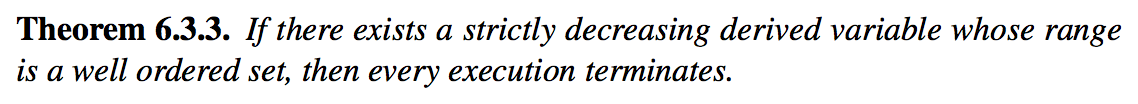This last section concludes the proof: in the step 2 we showed that a derived variable 𝑣 is a strictly decreasing derived variable whose range is a well ordered set ℕ + 𝔽 so we conclude that for any sequence of steps that robot can do it will eventually end up being in (0, 0).

## Geometric interpretation

Let’s consider that the robot is moving on 10 x 10 quadrant. By definition of 𝑣 we have:

𝑣(𝑥, 𝑦) := 𝑦 + 𝑥 / (𝑥 + 1)

therefore we get the following table of values:

``````(9 + 0/1)    (9 + 1/2)    (9 + 2/3)   ... (9 + 9/10)  # 9 ≤ y + x / (x + 1) ≤ 10
...                     ...                      ...
(2 + 0/1)    (2 + 1/2)    (2 + 2/3)   ... (2 + 9/10)  # 2 ≤ y + x / (x + 1) ≤ 3
(1 + 0/1)    (1 + 1/2)    (1 + 2/3)   ... (1 + 9/10)  # 1 ≤ y + x / (x + 1) ≤ 2
(0 + 0/1)    (0 + 1/2)    (0 + 2/3)   ... (0 + 9/10)  # 0 ≤ y + x / (x + 1) ≤ 1
``````

We see that the rows form buckets of values constrained within the range of [𝑦, 𝑦 + 1], for each { 𝑦 ∈ ℕ | 0 ≤ 𝑦 ≤ 10 }:

𝑦 ≤ 𝑦 + 𝑥 / (𝑥 + 1) < 𝑦 + 1 (Observation 1)

for example 2 ≤ (2 + 0/1) < (2 + 1/2) < (2 + 2/3) < … < (2 + 9/10) < 3.

Also, we see that columns form buckets of points so that the difference between the values of any two adjacent points equals to 1, for example:

(2 + 2/3) - (1 + 2/3) = 1 (Observation 2)

Observation 3. Sequences from the vertical buckets decrease with a rate of 1 per row and the sequences from the horizontal buckets decrease with a rate strictly less than 1 per column so vertical sequences decrease faster.

When robot jumps to the West it changes the column bucket which means that the 𝑦 stays the same, but 𝑥 changes to 𝑥 - 1 , for example:

(5, 5, 5 + 5/6) ⟶ (4, 5, 5 + 4/5) # 5 + 5 / 6 > 5 + 4/5

When robot jumps to the South East it changes the column and row buckets which means that

(5, 5, 5 + 5/6) ⟶ (𝑘, 4, 4 + (𝑘) / (𝑘 + 1)), 5 ≤ k ≤ 10

Along 𝑥-axis, Robot can jump to a 𝑘 coordinate as far as the right boundary of a quadrant, however its jump also changes the horizontal bucket 𝑦 - 1 which means that this jump to South is always a stronger contributor to the value 𝑣 than an arbitrary jump to the East (this is obvious from the observations 1, 2 and 3).

Now is clear that after each of these two moves derived value 𝑣 becomes smaller than (5, 5).

### Plots

Let’s look at the geometric interpretation of this function:

𝑓(𝑥, 𝑦) = 𝑦 + 𝑥 / (𝑥 + 1)

This is a three-dimensional function 𝑧 = 𝑦 + 𝑥 / (𝑥 + 1) so we will need a 3D plot to visualize this function: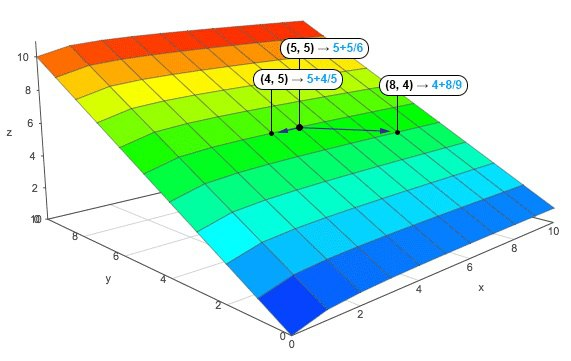Let’s assume that the robot is jumping from (5, 5) coordinate to either (4, 5) with its “unit distance West move” or (8, 4) with its “unit distance South combined with an arbitrary jump”.

When I look at the annotated plot above, I see a good analogy: the plot represents a fragment of a mountain which the robot is trying climb up: values of 𝑧 like (5 + 5/6) or (4 + 8/9) represent the altitudes corresponding to the coordinates (𝑥, 𝑦) of robot’s positions.

The drama of this robot, however, is that it can only move down the hill so it can never climb up. The altitude of its position is always decreasing and since it jumps on a limited 10 x 10 quadrant and the points are nonnegative integer numbers, the termination claim that we proved asserts that sooner or later robot will reach coordinate (0, 0) and get stuck.

The following plots demonstrate how the function 𝑓(𝑥, 𝑦) = 𝑦 + 𝑥 / (𝑥 + 1) looks like when it is not discrete but more continuous. These plots demonstrate it even better that the function is decreasing to 0 both along 𝑥 and 𝑦 axes.

3D Plot by Wolfram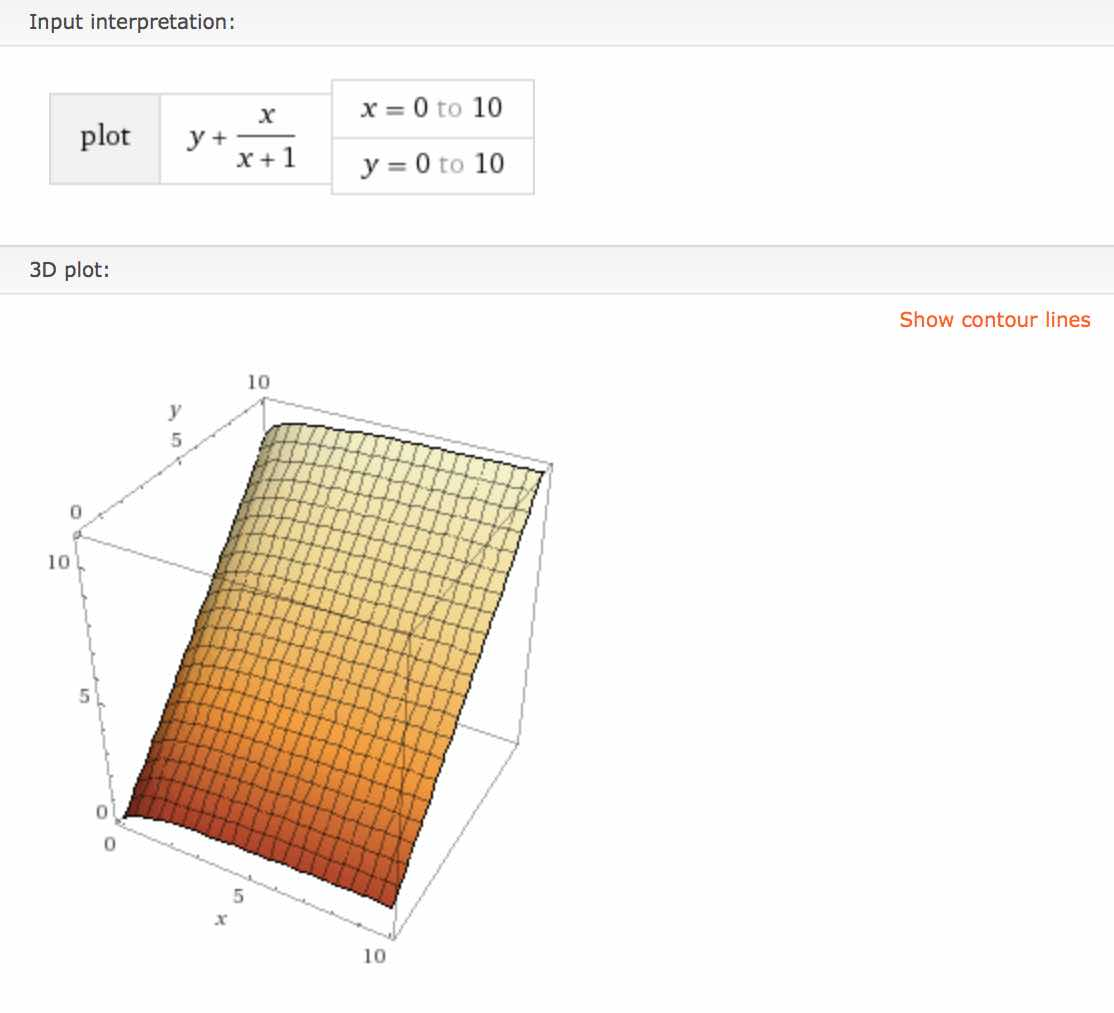3D Plot by Academo (Front)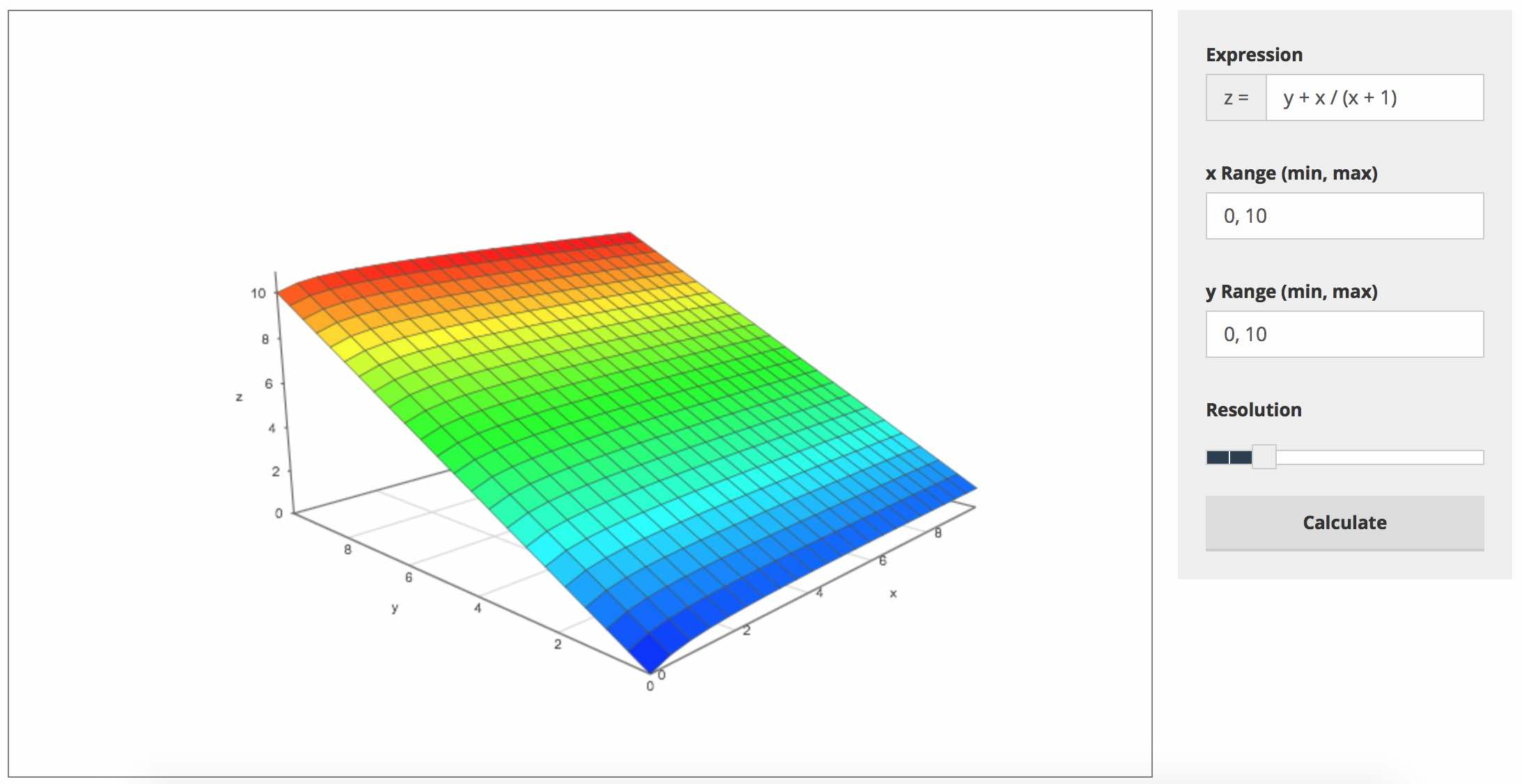3D Plot by Academo (Back)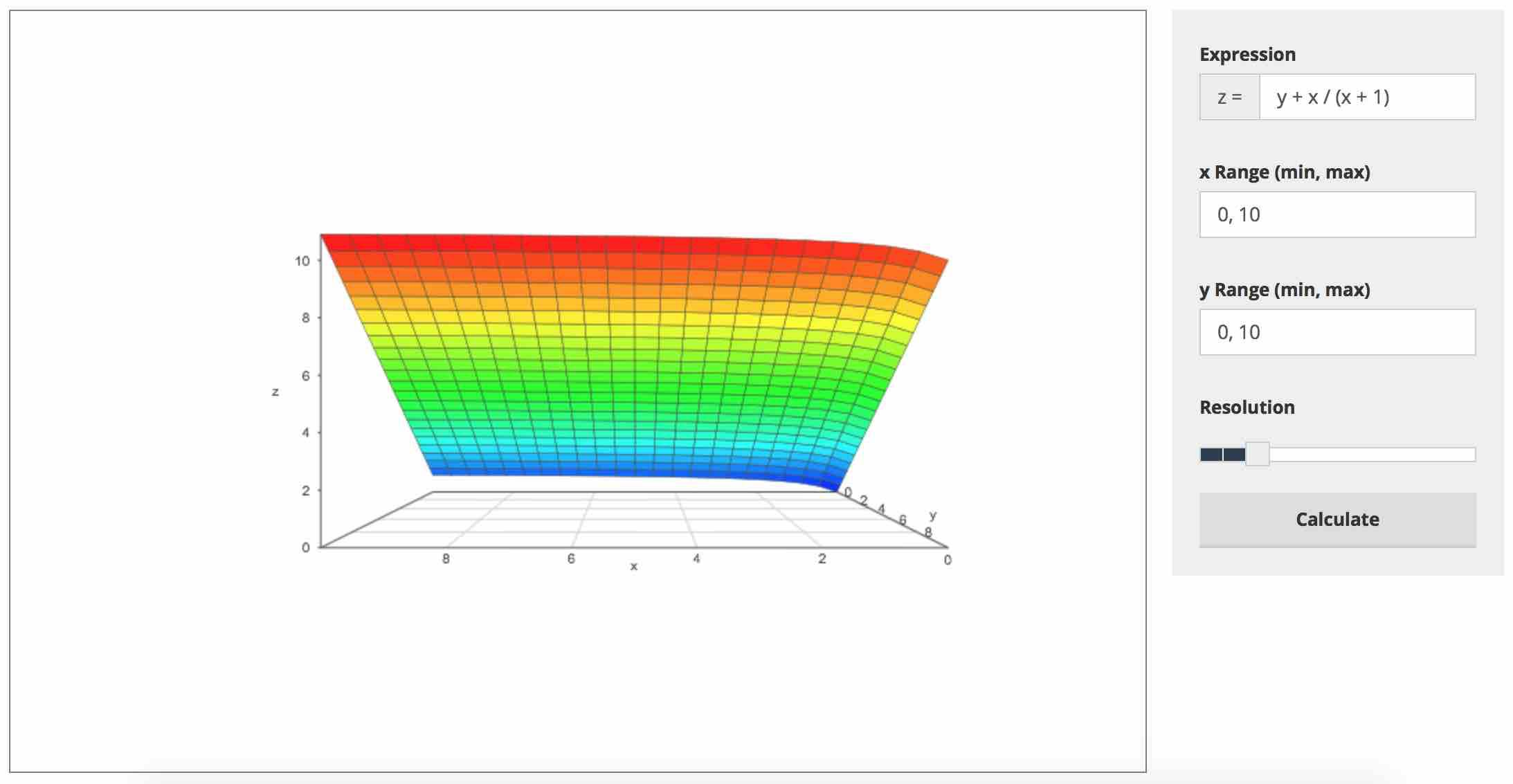## Credits

I am working with this proof because it is the material for the Week 2 of the course: “Formal Software Verification” . It is unlikely that I would have encountered this problem otherwise.

I used to two online services: Wolfram|Alpha  and Academo.org  to draw the plots. I used the amazing Academo service to create the annotated plot which helped me to visualize and understand the geometric interpretation of the proof.